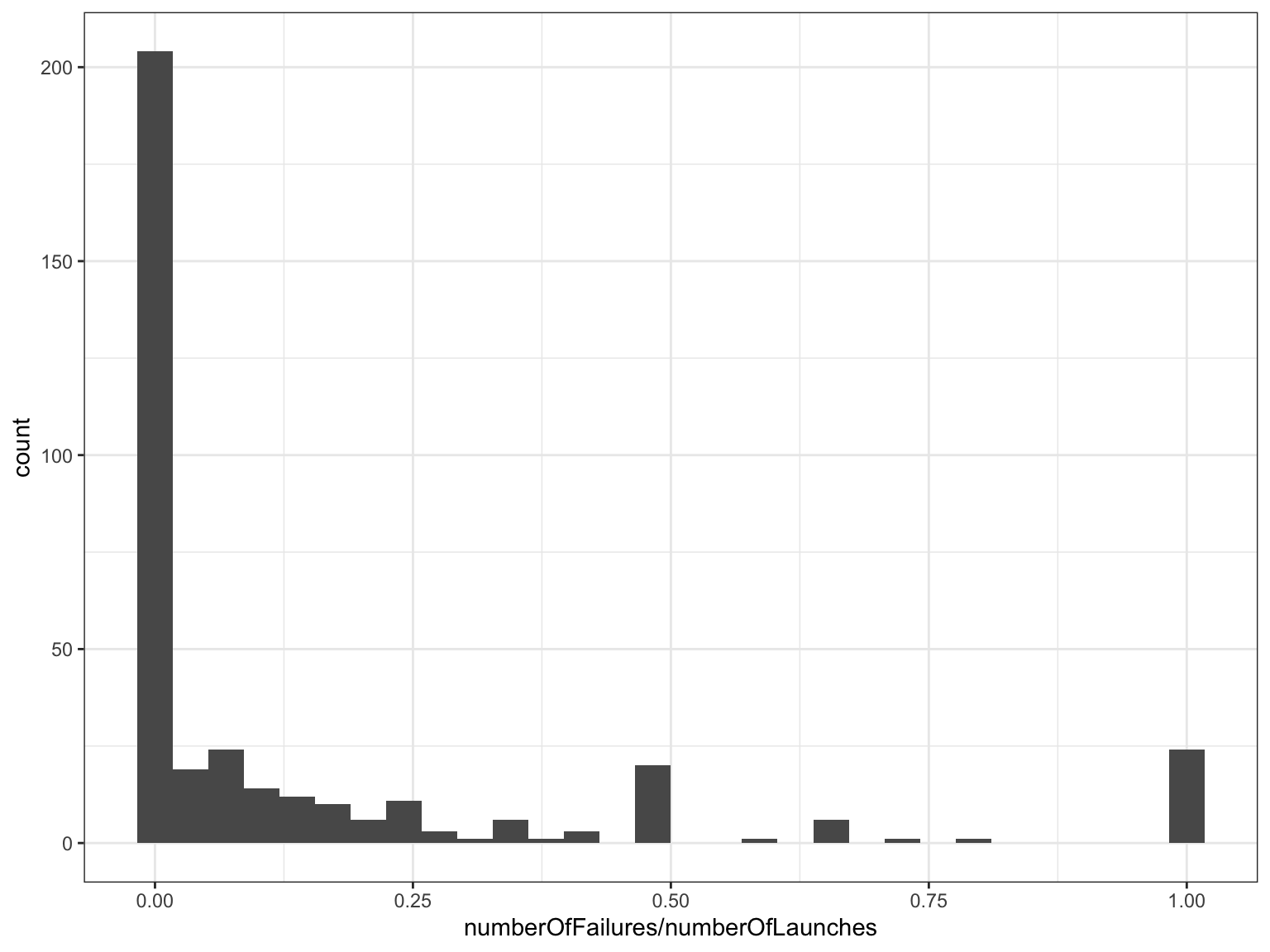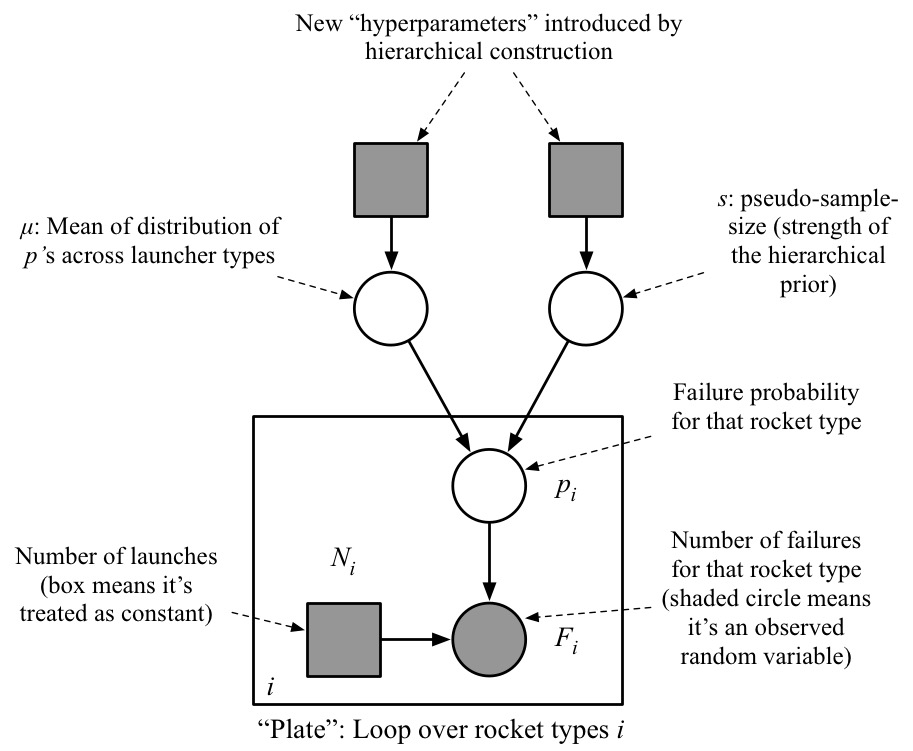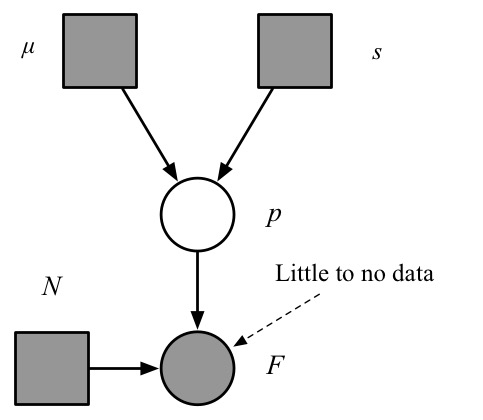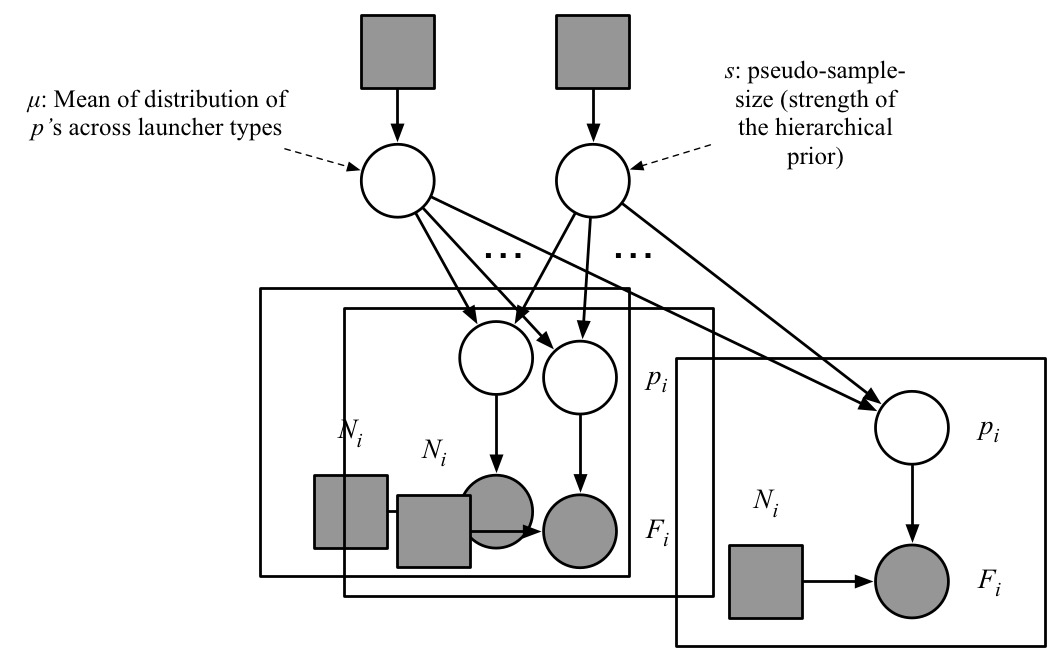# Hierarchical model: motivation

package ex.be

model LaunchPrediction {

random RealVar failureProbability ?: latentReal
random IntVar
launch1 ?: latentInt,
launch2 ?: latentInt,
launch3 ?: latentInt,
nextLaunch ?: latentInt

laws {
launch1 | failureProbability ~ Bernoulli(failureProbability)
launch2 | failureProbability ~ Bernoulli(failureProbability)
launch3 | failureProbability ~ Bernoulli(failureProbability)

nextLaunch | failureProbability ~ Bernoulli(failureProbability)

failureProbability ~ ContinuousUniform(0, 1)
}
}
• We used a uniform prior on the parameter failureProbability

### Poll: Do you know of other reasonable choices of prior here?

1. No
2. Yes, the Gamma distribution
3. Yes, the Beta distribution
4. Yes, the Weibull distribution
5. Yes, the Beta distribution and many others

# Choosing prior

### General approach

Often, the choice of prior is approached in two stages

• First, pick a distribution family (in our example, let us pick say Beta$$(\cdot, \cdot)$$, a common starting point for distribution with support $$[0, 1]$$)
• Then, pick one member of this family (e.g. Beta$$(1, 2)$$, but how can we do this?)

### When does the choice of prior matter?

• When data is large, posterior tends to be less sensitive to specification of the prior
• Will discuss theoretical reason for this soon: Bernstein von-Mises theorem
• Not always true (e.g. weakly identifiable model)
• When data is small, posterior tends to be more sensitive to specification of the prior
• Extreme example: for a maiden flight, what will the posterior look like?

### Strategies for constructing priors

• “Informative priors”: use expert knowledge
• e.g.: when we build rockets, there is a lot of quality control, so it would be surprising to see very high values for the parameter failureProbability
• bag of trick (Robert, Sections 3.1-3.3), will go over some techniques later, but none are completely satisfactory
• “Non-informative priors”: use properties of the likelihood to determine prior (Robert, Sections 3.5)
• not automated, case-by-case mathematical derivation often intractable
• room for an ambitious project here potentially
• Today: side-step these issues thanks to Hierarchical models
• We will not remove the need for prior choice, instead we will decrease sensitivity of the posterior to these choices

# Hierarchical models

Key idea: use “side data”" to inform the prior

• For example: success/fail launch data from other other types of rockets
• Can we use the full rocket launch data provided to inform prediction for a single rocket type of interest?
data <- read.csv("failure_counts.csv")
data %>%
knitr::kable(floating.environment="sidewaystable")
LV.Type numberOfLaunches numberOfFailures
Aerobee 1 0
Angara A5 1 0
Antares 110 2 0
Antares 120 2 0
Antares 130 1 1
Antares 230 1 0

# How to (badly) use side data

First try

• Merge all the data?
• I.e. just sum the columns in the data
LV.Type numberOfLaunches numberOfFailures
Aerobee 1 0
Angara A5 1 0
Antares 110 2 0
Antares 120 2 0
Antares 130 1 1
Antares 230 1 0

• Consider: adding to the dataset one more rocket type, launched successfully 10,000 times…
• Is there a better way?

# Towards an improved way to use side data

• Background: “mean-pseudo-sample-size” reparameterization of the Beta distribution
• A reparametrization is a different labelling of a family such that you can go back and forth between the two labellings
• Consider $\alpha = \mu s, \;\; \beta = (1 - \mu) s$ where $$\mu \in (0, 1)$$, $$s > 0$$.
• Interpretation:
• $$\mu$$: mean of the Beta
• $$s$$: measure of “peakiness” of the density, higher $$s$$ corresponds to more peaked; roughly, $$s \sim$$ number of data points that would make the posterior peaked like that.
• Why we did this reparameterization?
• Now it should be more intuitive how we could go about using side data to inform at least $$\mu$$
• Ideas?

# An improved way to use side data (not quite full Bayesian yet!)

• Estimate $$p_i$$ for each type of rocket
• Fit a distribution
• Use this distribution as the prior on $$\mu$$
• Weakness? Hint: what are the bumps at 1/2 and 1?
• Also: less clear how to do this with the pseudo-sample-size $$s$$
counts <- read.csv("failure_counts.csv")
ggplot(counts, aes(x = numberOfFailures / numberOfLaunches)) +
geom_histogram()# Solution: go fully Bayesian

Recall:

1. Construct a probability model including
• random variables for what we will measure/observe
• random variables for the unknown quantities
• those we are interested in (“parameters”, “predictions”)
• others that just help us formulate the problem (“nuisance”, “random effects”).
2. Compute the posterior distribution conditionally on the actual data at hand
3. Use the posterior distribution to:
• make prediction (point estimate)
• estimate uncertainty (credible intervals)
• make a decisionIn our case: just make $$\mu$$ and $$s$$ random! (or equivalently, $$\alpha$$ and $$\beta$$)

• Share these two “global parameters” across all launch types $p_i | \mu, s \sim {\text{Beta}}(\mu s, (1 - \mu) s)$
• We still need to put prior on $$\mu$$ and $$s$$
• But you should expect this prior choice to be less sensitive. Why? Hint: look at the number of outgoing edges in the graphical model. Compare with number of outgoing edges for the “maiden flight” (see next slide)
• Example: $$\mu \sim {\text{Beta}}(1,1) = {\text{Unif}}(0, 1)$$, $$s \sim \text{Exponential}(1/10000)$$ (why such a small value? Hint: mean vs. rate parameter)

# New higher-level hyperparameters = new problems? No we are probably ok!

It seems we have introduced new problems as now we again have hyperparameters, namely those for the priors on $$\mu$$ and $$s$$. Here we picked $$\mu \sim {\text{Beta}}(1,1) = {\text{Unif}}(0, 1)$$, $$s \sim \text{Exponential}(1/10000)$$

Key point: yes, but now we are less sensitive to these choices!

Why? Heuristic: say you have a random variable connected to some hyper-parameters (grey squares) and random variables connected to data (circles)

• If most of the connections are hyper-parameters: will probably be sensitive
• If there are many more connections to random variables compared to hyper-parameters: will probably be insensitive

Before going hierarchical: for maiden/early flights we hadAfter going hierarchical:• I didn’t show you the full data I scraped on rocket launches. See below
• Can we exploit the full structure?
• E.g. CC: Cape Canaveral Launch (american); NIIP Scientific Research Test Range, Russian: Nauchno-Issledovatel’skii Ispytatel’nyi Poligon
• For a relatively newer type of rocket, say Falcon Heavy, should we share parameters across all $$p_i$$ or maybe just the other american rocket types?
• If we settle on the latter, what if later we want to do a prediction for another country?
full <- read.csv("processed.csv")
 "    X  X..Launch    Launch.Date..UTC.      COSPAR         PL.Name                        Orig.PL.Name                SATCAT   LV.Type                 LV.S.N            Site                              Suc   Ref                     Suc_bin  Family           Space.Port    Year   Launch.Index"
 "-----  -----------  ---------------------  -------------  -----------------------------  --------------------------  -------  ----------------------  ----------------  --------------------------------  ----  ---------------------  --------  ---------------  -----------  -----  -------------"
 "    1  1957 ALP     1957 Oct  4 1928:34    1957 ALP 2     1-y ISZ                        PS-1                        S00002   Sputnik 8K71PS          M1-PS             NIIP-5   LC1                      S     Energiya                      1  Sputnik          NIIP          1957              1"
 "    2  1957-U01     1957 Oct 17 0505       1957-U01       USAF 88 Charge A               Poulter Pellet              A08258   Aerobee                 USAF 88           HADC     A                        S     EngSci1.58                    1  Aerobee          HADC          1957              1"
 "    3  1957 BET     1957 Nov  3 0230:42    1957 BET 1     2-y ISZ                        PS-2                        S00003   Sputnik 8K71PS          M1-2PS            NIIP-5   LC1                      S     Grahn-WWW                     1  Sputnik          NIIP          1957              2"
 "    4  1957-F01     1957 Dec  6 1644:35    1957-F01       Vanguard                       Vanguard Test Satellite     F00002   Vanguard                TV-3              CC       LC18A                    F     Vang-ER9948                   0  Vanguard         CC            1957              1"

# Taller hierarchies

• Natural idea: recurse! Think about it as the stories of a tall building:
• Foundations: launch data organized by type of rocket
• First floor of the model: parameters for each type of rocket $$p_i$$ $p_i | \mu_{c(i)}, s_{c(i)} \sim {\text{Beta}}(\mu_{c(i)} s_{c(i)}, (1 - \mu_{c(i)}) s_{c(i)})$ where $$c(i)$$ is the country for rocket type $$i$$
• Second floor: country-specific parameters, $$\mu_c$$, $$s_c$$ $\mu_c | \mu, s \sim {\text{Beta}}(\mu s, (1 - \mu s))$ $s_{c(i)} | \alpha, \beta \sim {\text{Gamma}}(\alpha, \beta)$
• Third floor: global parameters $$\mu, s, \alpha, \beta$$
• In principle, could build as many floors in that hierarchy as factors in the dataset.
• In theory could go taller. Why we don’t usually do that in practice? Hint: again, draw graphical model.
• Here we assumed that the hierachical structure is known
• Some work investigate inferring that structure, google “Bayesian hierarchical clustering” to get one flavour of this

# Exercise

Review the concepts in this topic by going over this exercise set: Hierarchical_models.html